Jdk1.8 HashMap实现原理详细介绍

更新时间：2016年12月22日 17:13:52   投稿：lqh我要评论

HashMap概述

HashMap是基于哈希表的Map接口的非同步实现。此实现提供所有可选的映射操作，并允许使用null值和null键。此类不保证映射的顺序，特别是它不保证该顺序恒久不变。

HashMap的数据结构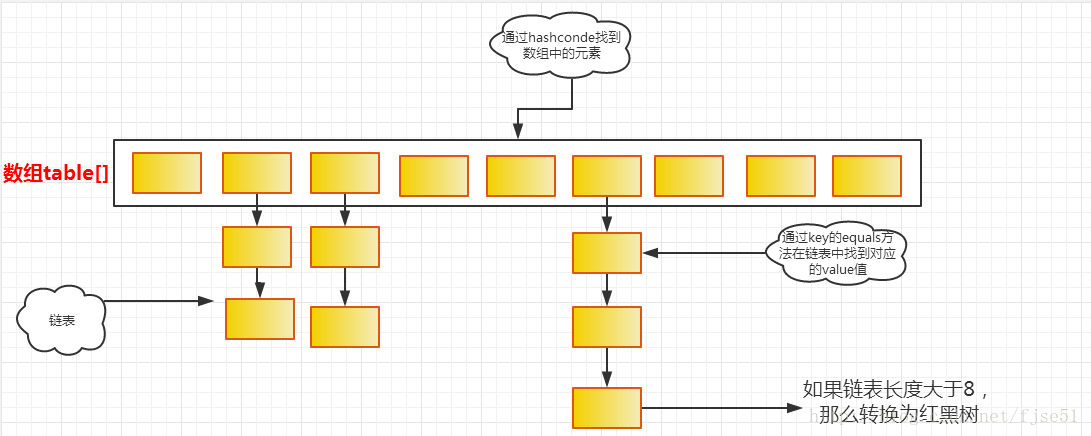*/
static class Node<K,V> implements Map.Entry<K,V> {
final int hash;//用于定位数组索引的位置
final K key;
V value;
Node<K,V> next;//链表的下一个Node

Node(int hash, K key, V value, Node<K,V> next) {
this.hash = hash;
this.key = key;
this.value = value;
this.next = next;
}

Node是HashMap的一个内部类，实现了Map.Entry接口，本质是就是一个映射(键值对)。

HashMap类中有一个非常重要的字段，就是 Node[] table，即哈希桶数组，明显它是一个Node的数组。

int threshold;    // 所能容纳的key-value对极限
final float loadFactor; // 负载因子
int modCount;
int size;

size这个字段其实很好理解，就是HashMap中实际存在的键值对数量。注意和table的长度length、容纳最大键值对数量threshold的区别。而modCount字段主要用来记录HashMap内部结构发生变化的次数，主要用于迭代的快速失败。强调一点，内部结构发生变化指的是结构发生变化，例如put新键值对，但是某个key对应的value值被覆盖不属于结构变化。

//方法一：
static final int hash(Object key) { //jdk1.8 & jdk1.7
int h;
// h = key.hashCode() 为第一步 取hashCode值
// h ^ (h >>> 16) 为第二步 高位参与运算
return (key == null) ? 0 : (h = key.hashCode()) ^ (h >>> 16);
}
//方法二：
static int indexFor(int h, int length) { //jdk1.7的源码，jdk1.8没有这个方法，但是实现原理一样的
return h & (length-1); //第三步 取模运算
}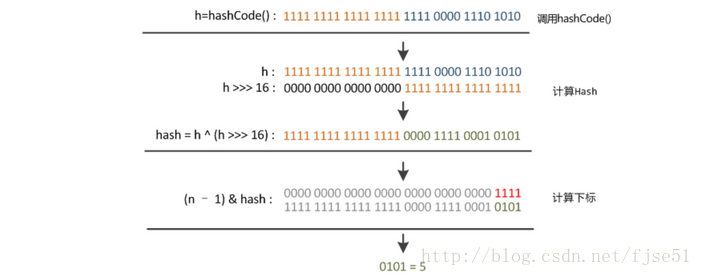HashMap的put方法实现

put函数大致的思路为：

1. 对key的hashCode()做hash，然后再计算index;
2. 如果没碰撞直接放到bucket里；
3. 如果碰撞了，以链表的形式存在buckets后；
4. 如果碰撞导致链表过长(大于等于TREEIFY_THRESHOLD)，就把链表转换成红黑树；
5. 如果节点已经存在就替换old value(保证key的唯一性)
6. 如果bucket满了(超过load factor*current capacity)，就要resize。

public V put(K key, V value) {

return putVal(hash(key), key, value, false, true);
}
/**
*生成hash的方法
*/
static final int hash(Object key) {
int h;
return (key == null) ? 0 : (h = key.hashCode()) ^ (h >>> 16);
}

final V putVal(int hash, K key, V value, boolean onlyIfAbsent,boolean evict) {
Node<K,V>[] tab; Node<K,V> p; int n, i;
//判断table是否为空，
if ((tab = table) == null || (n = tab.length) == 0)
n = (tab = resize()).length;//创建一个新的table数组，并且获取该数组的长度
//根据键值key计算hash值得到插入的数组索引i，如果table[i]==null，直接新建节点添加
if ((p = tab[i = (n - 1) & hash]) == null)
tab[i] = newNode(hash, key, value, null);
else {//如果对应的节点存在
Node<K,V> e; K k;
//判断table[i]的首个元素是否和key一样，如果相同直接覆盖value
if (p.hash == hash &&
((k = p.key) == key || (key != null && key.equals(k))))
e = p;
//判断table[i] 是否为treeNode，即table[i] 是否是红黑树，如果是红黑树，则直接在树中插入键值对
else if (p instanceof TreeNode)
e = ((TreeNode<K,V>)p).putTreeVal(this, tab, hash, key, value);
// 该链为链表
else {
//遍历table[i]，判断链表长度是否大于TREEIFY_THRESHOLD(默认值为8)，大于8的话把链表转换为红黑树，在红黑树中执行插入操作，否则进行链表的插入操作；遍历过程中若发现key已经存在直接覆盖value即可；
for (int binCount = 0; ; ++binCount) {
if ((e = p.next) == null) {
p.next = newNode(hash, key, value, null);
if (binCount >= TREEIFY_THRESHOLD - 1) // -1 for 1st
treeifyBin(tab, hash);
break;
}
if (e.hash == hash &&
((k = e.key) == key || (key != null && key.equals(k))))
break;
p = e;
}
}
// 写入
if (e != null) { // existing mapping for key
V oldValue = e.value;
if (!onlyIfAbsent || oldValue == null)
e.value = value;
afterNodeAccess(e);
return oldValue;
}
}
++modCount;
// 插入成功后，判断实际存在的键值对数量size是否超多了最大容量threshold，如果超过，进行扩容
if (++size > threshold)
resize();
afterNodeInsertion(evict);
return null;
}

HashMap的get方法实现

1.bucket里的第一个节点，直接命中；

2.如果有冲突，则通过key.equals(k)去查找对应的entry

public V get(Object key) {
Node<K,V> e;
return (e = getNode(hash(key), key)) == null ? null : e.value;
}

final Node<K,V> getNode(int hash, Object key) {
Node<K,V>[] tab; Node<K,V> first, e; int n; K k;
if ((tab = table) != null && (n = tab.length) > 0 &&
(first = tab[(n - 1) & hash]) != null) {
// 直接命中
if (first.hash == hash && // 每次都是校验第一个node
((k = first.key) == key || (key != null && key.equals(k))))
return first;
// 未命中
if ((e = first.next) != null) {
// 在树中获取
if (first instanceof TreeNode)
return ((TreeNode<K,V>)first).getTreeNode(hash, key);
// 在链表中获取
do {
if (e.hash == hash &&
((k = e.key) == key || (key != null && key.equals(k))))
return e;
} while ((e = e.next) != null);
}
}
return null;
}

void resize(int newCapacity) { //传入新的容量
Entry[] oldTable = table; //引用扩容前的Entry数组
int oldCapacity = oldTable.length;
if (oldCapacity == MAXIMUM_CAPACITY) { //扩容前的数组大小如果已经达到最大(2^30)了
threshold = Integer.MAX_VALUE; //修改阈值为int的最大值(2^31-1)，这样以后就不会扩容了
return;
}

Entry[] newTable = new Entry[newCapacity]; //初始化一个新的Entry数组
transfer(newTable);       //！！将数据转移到新的Entry数组里
table = newTable;       //HashMap的table属性引用新的Entry数组
threshold = (int)(newCapacity * loadFactor);//修改阈值
}

void transfer(Entry[] newTable) {
Entry[] src = table;     //src引用了旧的Entry数组
int newCapacity = newTable.length;
for (int j = 0; j < src.length; j++) { //遍历旧的Entry数组
Entry<K,V> e = src[j];    //取得旧Entry数组的每个元素
if (e != null) {
src[j] = null;//释放旧Entry数组的对象引用（for循环后，旧的Entry数组不再引用任何对象）
do {
Entry<K,V> next = e.next;
int i = indexFor(e.hash, newCapacity); //！！重新计算每个元素在数组中的位置
e.next = newTable[i]; //标记
newTable[i] = e;  //将元素放在数组上
e = next;    //访问下一个Entry链上的元素
} while (e != null);
}
}
}

newTable[i]的引用赋给了e.next，也就是使用了单链表的头插入方式，同一位置上新元素总会被放在链表的头部位置；这样先放在一个索引上的元素终会被放到Entry链的尾部(如果发生了hash冲突的话），这一点和Jdk1.8有区别，下文详解。在旧数组中同一条Entry链上的元素，通过重新计算索引位置后，有可能被放到了新数组的不同位置上。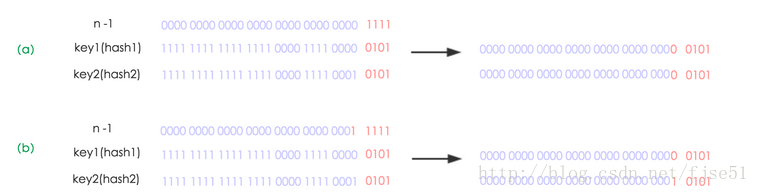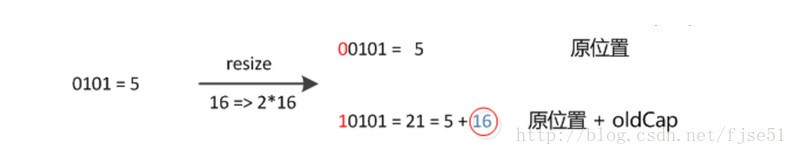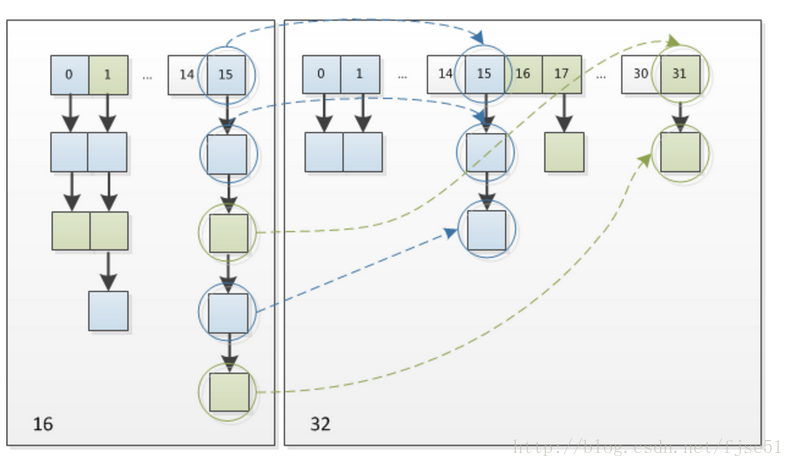final Node<K,V>[] resize() {
Node<K,V>[] oldTab = table;
int oldCap = (oldTab == null) ? 0 : oldTab.length;
int oldThr = threshold;
int newCap, newThr = 0;
if (oldCap > 0) {
// 超过最大值就不再扩充了，就只好随你碰撞去吧
if (oldCap >= MAXIMUM_CAPACITY) {
threshold = Integer.MAX_VALUE;
return oldTab;
}
// 没超过最大值，就扩充为原来的2倍
else if ((newCap = oldCap << 1) < MAXIMUM_CAPACITY &&
oldCap >= DEFAULT_INITIAL_CAPACITY)
newThr = oldThr << 1; // double threshold
}
else if (oldThr > 0) // initial capacity was placed in threshold
newCap = oldThr;
else {    // zero initial threshold signifies using defaults
newCap = DEFAULT_INITIAL_CAPACITY;
newThr = (int)(DEFAULT_LOAD_FACTOR * DEFAULT_INITIAL_CAPACITY);
}
// 计算新的resize上限
if (newThr == 0) {

float ft = (float)newCap * loadFactor;
newThr = (newCap < MAXIMUM_CAPACITY && ft < (float)MAXIMUM_CAPACITY ?
(int)ft : Integer.MAX_VALUE);
}
threshold = newThr;
@SuppressWarnings({"rawtypes","unchecked"})
Node<K,V>[] newTab = (Node<K,V>[])new Node[newCap];
table = newTab;
if (oldTab != null) {
// 把每个bucket都移动到新的buckets中
for (int j = 0; j < oldCap; ++j) {
Node<K,V> e;
if ((e = oldTab[j]) != null) {
oldTab[j] = null;
if (e.next == null)
newTab[e.hash & (newCap - 1)] = e;
else if (e instanceof TreeNode)
((TreeNode<K,V>)e).split(this, newTab, j, oldCap);
else { // preserve order
Node<K,V> loHead = null, loTail = null;
Node<K,V> hiHead = null, hiTail = null;
Node<K,V> next;
do {
next = e.next;
// 原索引
if ((e.hash & oldCap) == 0) {
if (loTail == null)
else
loTail.next = e;
loTail = e;
}
// 原索引+oldCap
else {
if (hiTail == null)
else
hiTail.next = e;
hiTail = e;
}
} while ((e = next) != null);
// 原索引放到bucket里
if (loTail != null) {
loTail.next = null;
}
// 原索引+oldCap放到bucket里
if (hiTail != null) {
hiTail.next = null;
newTab[j + oldCap] = hiHead;
}
}
}
}
}
return newTab;
}

1.什么时候会使用HashMap？他有什么特点？

2.你知道HashMap的工作原理吗？

3.你知道get和put的原理吗？equals()和hashCode()的都有什么作用？

4.你知道hash的实现吗？为什么要这样实现？

iterator()时顺着哈希桶数组来遍历，看起来是个乱序。

6.当两个对象的hashcode相同会发生什么？

7.如果两个键的hashcode相同，你如何获取值对象？

9.你了解重新调整HashMap大小存在什么问题吗？

10.为什么String, Interger这样的wrapper类适合作为键？